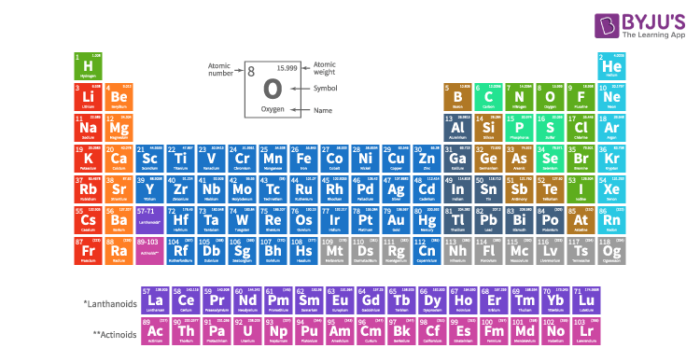Jet Set Go! All about Aeroplanes Jet Set Go! All about Aeroplanes

# Periodic Properties Of Elements and Their Significance

## Periodic properties of elements

The basic law governing modern periodic table states that the properties of elements are periodic functions of their atomic number. These properties reappear at regular intervals or follow a particular trend at regular intervals. This phenomenon is known as the periodicity of elements.

The periodic properties of elements occur due to the recurrence of similar electronic configuration that is having the same number of electrons in the outermost orbit. In a particular group, the number of valence electrons remains the same. On the other hand, the number of valence electrons increases, as we move from left to right across a period. The chemical property of an element depends on the number of electrons in the valence shell.## Explanation for periodic properties

The periodic properties of an element depend on valency and number of shells in an atom. As we move down a group the number of shell increases successively such that the number of the shell of an element is equal to the number of periods to which it belongs. As we move across a period, the number of shell remains the same. For example, elements of the second period have two shells.

The combining capacity of an atom is known as its valency. It is equal to the number of electrons that an atom can accept or donate in order to complete its octet. As we move down a group, the number of electrons in the valence shell remains the same. Hence, the valency of a group is constant. Valency depends on the number of electrons in the outermost shell of an atom. If the number of electrons is 1, 2, 3, 4 then the respective valences will be 1, 2, 3, 4. If the number of electrons in the outermost shell will be 5, 6, 7 then the valency will be 8 – 5 = 3, 8 – 6 = 2 and 8 – 7 = 1. Valency is the combining capacity of an atom hence will always have a positive value and largely affects the periodic properties.

In a period, the number of electrons increases from left to right. As a result, the number of electrons needed to complete the octet also changes. Hence, the valency successively increases to four in group 14 and then subsequently decreases to 1 in group 17.

We have seen the periodic properties of elements in the periodic table. To know more, register with BYJU’S and download our app.